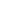# The simplest CPU Scheduling algorithm is _____.

a) SJF Scheduling algorithm
b) Round robin Scheduling algorithm
c) Multilevel Scheduling algorithm
d) FCFS Scheduling algorithm
SSC CHSL-2015

 FCFS Scheduling algorithm. First-Come-First-Served algorithm is the simplest scheduling algorithm is the simplest scheduling algorithm.
Share :
+
Next
« Prev
Prev
Next »